# Working Time Calculation Based on Timesheets

In the office, a special machine record the time when you start working (clock in office) and when you finish working (clock out of office). We can calculate the total working time by subtracting the end working time from the start working time.

If we also take out the lunch break, we have to subtract the lunch break from the total working time we get. See example below, we calculate effective working hours with the help of Excel MOD function, launch break is “thrown away”.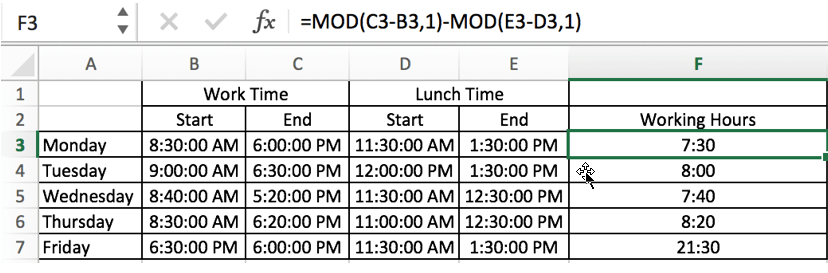Notes:

Before creating the formula to calculate the working time, we should first set the number format to “Time”.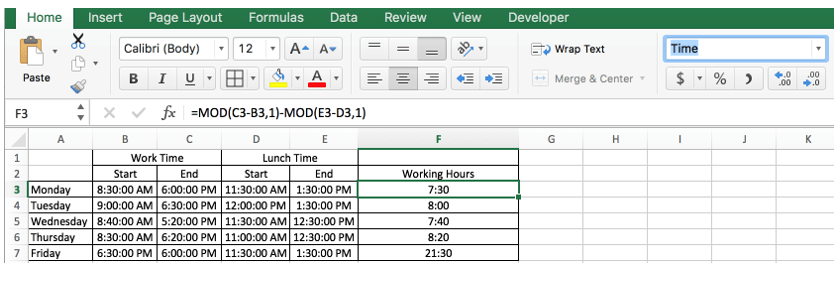## FORMULA

In this example, the formula is:

`=MOD(C3-B3,1)-MOD(E3-D3,1)`

## EXPLANATION

Here, you may wonder why we use the MOD function instead of just using the simple addition and subtraction operations. Firstly, let’s introduce Excel MOD function.

MOD function lists in Excel Formulas->Math & Trig functions.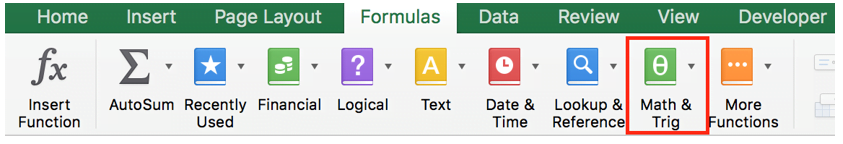MOD function returns the remainder of two numbers in a division operation.

Syntax:

`=MOD (number, divisor)`

For example, MOD(5,3)=2.

In the first MOD function:

#number: C3-B3

Subtract the start working time from the end working time, then we can get “total working hours”.

#divisor: 1

In the second MOD function:

#number: E3-D3

Subtract the start of the lunch break from the end of the lunch break to get “lunch break time”.

#divisor: 1

In this example, on Friday, the start time is Thursday 6:30:00 PM, but the end time is Friday 6:00:00 PM (cross midnight). If we ignore the day of the week, then the start time is later than the end time, and without the MOD function, Excel would return an error if it applied only subtraction operations. To cover the “across midnight” case (start time later than the end time, but lasting no more than 24 hours), we set the divisor argument to “1” to avoid getting a negative value. That’s why we apply Excel MOD function here to calculate working hours.

Take Friday as an example to calculate the working hours.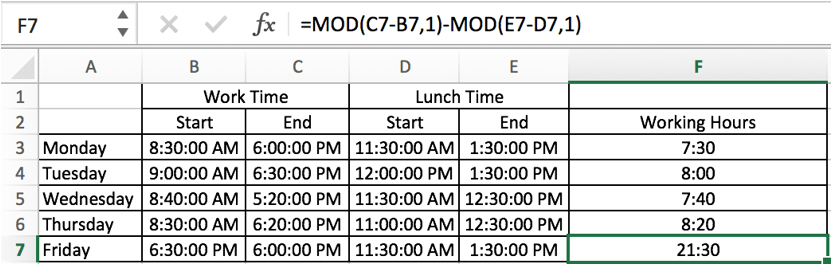Total 21 hours and 30 minutes are displayed after running the formula.

If we just apply subtraction, the number cannot be shown in “Time” format properly.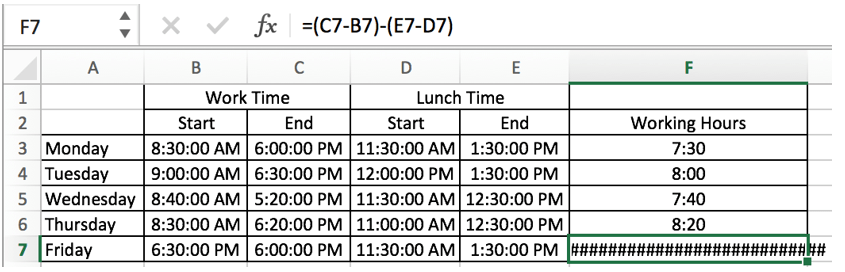If we set number format to “General”, a negative number is displayed.In the example, the formula =MOD(C3-B3,1)-MOD(E3-D3,1) is equivalent to “total working hours” minus “lunch break”. So the actual working time is calculated correctly by this formula.

### Related Functions

• Excel MOD function
the Excel MOD function returns the remainder of two numbers after division. So you can use the MOD function to get the remainder after a number is divided by a divisor in Excel. The syntax of the MOD function is as below:=MOD (number, divisor)….

Related Posts

Calculating Average Of The Numbers

Are you weary of investing a lot of time and effort in manually calculating the average of the numbers by including or excluding 0 and calculating the average of the top 3 scores? Then congratulations because you have just landed ...

Add Minutes to Time in Excel

Adding whole hours or decimal minutes to a given time in Excel is a fairly simple process. You can do this by using the Start_time+TIME(0,minutes,0) and Start_time+minutes/1440 functions. In this add minutes to time guide, we will show you how ...

Add Hours To Time in Excel

Adding whole hours or decimal hours to a given time in Excel is a fairly simple process. You can do this by using the Start_time+TIME(hours,0,0) and Start_time+hours/24 functions. In this add hours to time guide, we will show you how ...

Extract or Filter Every Nth Row

Assume that you're in a situation where you need to filter every nth row from a list having a few values. I'm pretty sure you'd choose to do it manually, which is also a great choice when you only have ...

How to Sum Every Nth Column in Excel

Sometimes for data listed in rows, we may want to sum data every Nth column, for example sum data only in ODD column or EVEN column (every 2 column). In our daily life, we may meet many cases like this. ...

Sidebar